# huidong

## 前言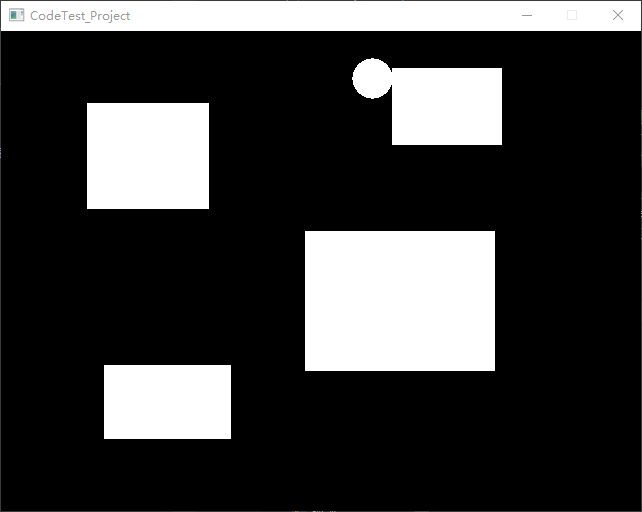https://learnopengl-cn.github.io/06%20In%20Practice/2D-Game/05%20Collisions/03%20Collision%20resolution/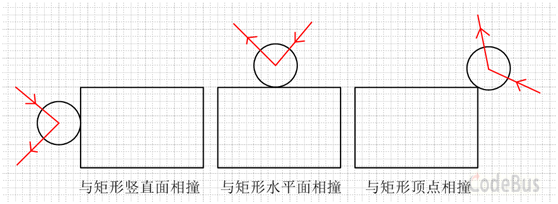## 背景设定

• 打篮球游戏，篮球与水平地面的碰撞（动圆与固定直线碰撞）
• 打台球游戏，动圆碰撞动圆（还有动圆碰撞固定的圆）
• 运动的圆与墙壁碰撞（动圆和固定矩形的碰撞）
• 运动的圆与运动的矩形碰撞
• 矩形之间的碰撞
• ……

• 动的打动的（运动的物体碰撞可以运动的物体，后者可以没有初速度）
• 动的打不动的（运动的物体碰撞不能运动的物体，比如和墙碰撞）

• 点与点的碰撞
• 点与线的碰撞
• 线与线的碰撞

## 理论分析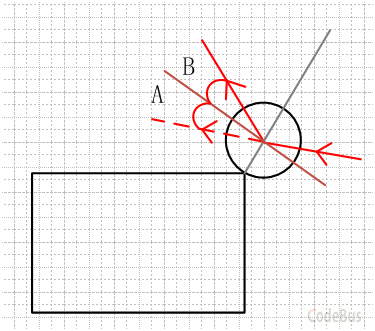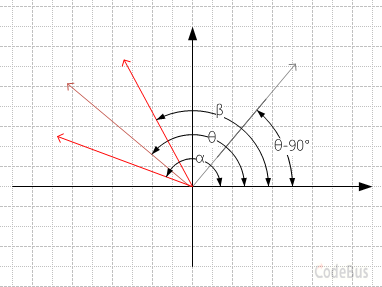α - θ = θ – β

β = 2θ – α

## 程序中的处理### 碰撞检测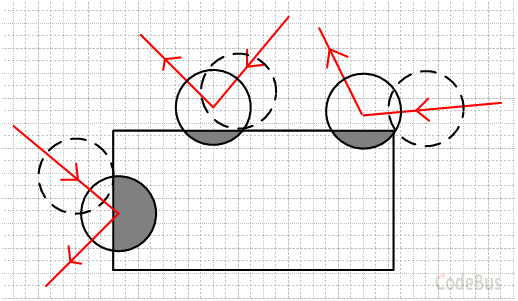``````// 获取点到矩形的最小距离
float GetDistance_PointToRect(float x, float y, RECT rct)
{
float x_rct, y_rct;	// 保存矩形内到目标点最近的点
if (x >= rct.left && x <= rct.right)
x_rct = x;
else
x_rct = (float)(fabsf(x - rct.left) < fabsf(x - rct.right) ? rct.left : rct.right);
if (y >= rct.top && y <= rct.bottom)
y_rct = y;
else
y_rct = (float)(fabsf(y - rct.top) < fabsf(y - rct.bottom) ? rct.top : rct.bottom);

float dx = x - x_rct;
float dy = y - y_rct;

return sqrtf(dx * dx + dy * dy);
}

``````

### 反弹处理1. 如果小球同时跨越了矩形的两条边界，那么就可以直接确定，只有这两条边界的公共顶点有可能发生顶点碰撞。
2. 如果小球只跨越了一条边界，那么我们可以认为这条边界所对应的两个顶点中，距离圆心更近的一个顶点才有可能发生顶点碰撞。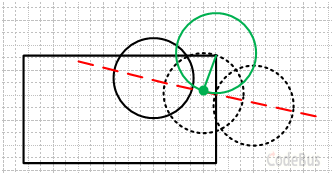``````// 根据圆的轨迹直线，获取圆与某点相切时的圆心坐标
// 返回是否存在相切
bool GetTangentCirclePoint(
float x0,		// 切点坐标
float y0,
float x1,		// 圆心轨迹直线上的一点（更早运动到的点）
float y1,
float x2,		// 圆心轨迹直线上的另一点（其实运动不到的点）
float y2,
float r,		// 圆半径
float* p_out_x,	// 输出圆心坐标
float* p_out_y
)
{
// 斜率不存在时
if (fabsf(x1 - x2) < 0.00001f)
{
// 计算相切时圆心与切点的竖直距离
float d2 = r * r - (x0 - x1) * (x0 - x1);
if (d2 < 0)
return false;
float d = sqrtf(d2);

// 求出两组解
float _y1 = y0 + d;
float _y2 = y0 - d;

// 保留离 (x1, y1) 更近的解
float _y_closer = fabsf(y1 - _y1) < fabsf(y1 - _y2) ? _y1 : _y2;

*p_out_x = x1;
*p_out_y = _y_closer;

return true;
}

// 圆心轨迹直线方程：y - y1 = (y2 - y1) / (x2 - x1) * (x - x1)
// 即：y = kx - kx1 + y1
// 圆的方程：(x - x0) ^ 2 + (y - y0) ^ 2 = r ^ 2
// 联立得二次函数，如下。

float k = (y2 - y1) / (x2 - x1);			// 直线斜率
float m = -k * x1 + y1 - y0;				// 部分常数
float a = k * k + 1;						// 二次函数的 abc 系数
float b = 2 * (k * m - x0);
float c = x0 * x0 + m * m - r * r;
float delta = b * b - 4 * a * c;			// 判别式
if (delta < 0)								// 无解
return false;
float sqrt_delta = sqrtf(delta);			// 判别式开根号
float _x1 = (-b + sqrt_delta) / (2 * a);	// 两个根
float _x2 = (-b - sqrt_delta) / (2 * a);

// 保留离 (x1, y1) 更近的解
float _x_closer = fabsf(x1 - _x1) < fabsf(x1 - _x2) ? _x1 : _x2;
float _y = k * _x_closer - k * x1 + y1;

*p_out_x = _x_closer;
*p_out_y = _y;

return true;
}

``````

β = 2θ – α

``````// 碰撞时的小球圆心坐标（即与顶点相切时的坐标）
float fCollisionX, fCollisionY;
if (!GetTangentCirclePoint(		// 获取相切时的圆心坐标
fVertex_X,		// 顶点坐标（前面已经求出）
fVertex_Y,
last_x,			// 上一帧的圆心坐标
last_y,
ball->x,		// 当前圆心坐标
ball->y,
&fCollisionX,	// 传入指针
&fCollisionY
))
{
// 没有相切，说明顶点碰撞不成立
//
// TODO ...
return;
}

// 如果是真的相切，则相切时矩形到圆心的最近距离应该等于小球半径
// 但如果此时小于半径，那么说明是假相切
if (GetDistance_PointToRect(fCollisionX, fCollisionY, rct) < g_nBallRadius * 0.98f /* 允许一点误差 */)
{
// 顶点碰撞不成立
//
// TODO ...
return;
}

// 计算碰撞时，小球圆心到碰撞点（顶点）的坐标差
float f_dx = fCollisionX - fVertex_X;
float f_dy = fCollisionY - fVertex_Y;

// 求反射面弧度

// 求法线弧度
float f_radianNormal = f_radianReflectingSurface + PI / 2 /* 或 - PI / 2 */;

// 求小球入射弧度

// 将小球速度沿法线对称，求得新的速度弧度

// 求反射速度

``````

``````// 修正小球坐标到相切时的坐标
ball->x = fCollisionX;
ball->y = fCollisionY;

``````### 特殊情况处理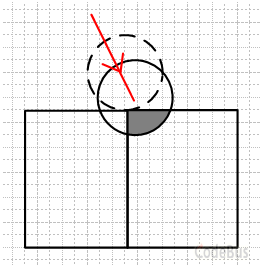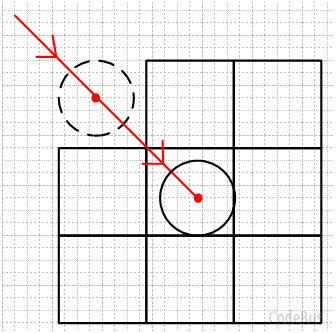``````// 得到小球上一帧的坐标
float last_x = (ball->x - ball->vx);
float last_y = (ball->y - ball->vy);

// 如果小球不慎进入砖块内部，此时应该回到上一帧，并使速度反向（同时加以扰动）
if (ball->x >= rct.left && ball->x <= rct.right
&& ball->y >= rct.top && ball->y <= rct.bottom)
{
ball->x = last_x;
ball->y = last_y;

// 如果上一帧仍然陷在里面
if (ball->x >= rct.left && ball->x <= rct.right
&& ball->y >= rct.top && ball->y <= rct.bottom)
{
// 强制弹出小球
if (ball->vx > 0 && left /* 左边界不与其他矩形相连 */)	// 小球穿越左边界
{
}
else if (ball->vx < 0 && right /* 右边界不与其他矩形相连 */)	// 小球穿越右边界
{
}
if (ball->vy > 0 && up /* 上边界不与其他矩形相连 */)	// 小球穿越上边界
{
}
else if (ball->vy < 0 && down /* 下边界不与其他矩形相连 */)	// 小球穿越下边界
{
}
}

ball->vx *= -1 + rand() % 10 / 1000.f;
ball->vy *= -1 + rand() % 10 / 1000.f;
}
``````

## 示例代码``````#include <graphics.h>
#include <math.h>
#include <time.h>

// 圆周率
#define PI			3.1415926f

// 窗口大小
#define WIDTH		640
#define HEIGHT		480

// 小球半径

// 小球速度
#define SPEED		10.f

// 小球
struct Ball
{
float x;
float y;
float vx;
float vy;
}ball;

// 获取点到矩形的最小距离
float GetDistance_PointToRect(float x, float y, RECT rct)
{
float x_rct, y_rct;	// 保存矩形内到目标点最近的点
if (x >= rct.left && x <= rct.right)
x_rct = x;
else
x_rct = (float)(fabsf(x - rct.left) < fabsf(x - rct.right) ? rct.left : rct.right);
if (y >= rct.top && y <= rct.bottom)
y_rct = y;
else
y_rct = (float)(fabsf(y - rct.top) < fabsf(y - rct.bottom) ? rct.top : rct.bottom);

float dx = x - x_rct;
float dy = y - y_rct;

return sqrtf(dx * dx + dy * dy);
}

// 根据圆的轨迹直线，获取圆与某点相切时的圆心坐标
// 返回是否存在相切
bool GetTangentCirclePoint(
float x0,		// 切点坐标
float y0,
float x1,		// 圆心轨迹直线上的一点（更早运动到的点）
float y1,
float x2,		// 圆心轨迹直线上的另一点（其实运动不到的点）
float y2,
float r,		// 圆半径
float* p_out_x,	// 输出圆心坐标
float* p_out_y
)
{
// 斜率不存在时
if (fabsf(x1 - x2) < 0.00001f)
{
// 计算相切时圆心与切点的竖直距离
float d2 = r * r - (x0 - x1) * (x0 - x1);
if (d2 < 0)
return false;
float d = sqrtf(d2);

// 求出两组解
float _y1 = y0 + d;
float _y2 = y0 - d;

// 保留离 (x1, y1) 更近的解
float _y_closer = fabsf(y1 - _y1) < fabsf(y1 - _y2) ? _y1 : _y2;

*p_out_x = x1;
*p_out_y = _y_closer;

return true;
}

// 圆心轨迹直线方程：y - y1 = (y2 - y1) / (x2 - x1) * (x - x1)
// 即：y = kx - kx1 + y1
// 圆的方程：(x - x0) ^ 2 + (y - y0) ^ 2 = r ^ 2
// 联立得二次函数，如下。

float k = (y2 - y1) / (x2 - x1);			// 直线斜率
float m = -k * x1 + y1 - y0;				// 部分常数
float a = k * k + 1;						// 二次函数的 abc 系数
float b = 2 * (k * m - x0);
float c = x0 * x0 + m * m - r * r;
float delta = b * b - 4 * a * c;			// 判别式
if (delta < 0)								// 无解
return false;
float sqrt_delta = sqrtf(delta);			// 判别式开根号
float _x1 = (-b + sqrt_delta) / (2 * a);	// 两个根
float _x2 = (-b - sqrt_delta) / (2 * a);

// 保留离 (x1, y1) 更近的解
float _x_closer = fabsf(x1 - _x1) < fabsf(x1 - _x2) ? _x1 : _x2;
float _y = k * _x_closer - k * x1 + y1;

*p_out_x = _x_closer;
*p_out_y = _y;

return true;
}

// 小球碰撞处理
// rct 碰撞箱区域
//
// 返回是否发生碰撞
bool BallCollisionProcess(RECT rct)
{
// 得到小球上一帧的坐标
float last_x = (ball.x - ball.vx);
float last_y = (ball.y - ball.vy);

bool is_collision = false;	// 是否发生碰撞

// 如果小球不慎进入砖块内部，此时应该回到上一帧，并使速度反向（同时加以扰动）
if (ball.x >= rct.left && ball.x <= rct.right
&& ball.y >= rct.top && ball.y <= rct.bottom)
{
ball.x = last_x;
ball.y = last_y;

// 如果上一帧仍然陷在里面
if (ball.x >= rct.left && ball.x <= rct.right
&& ball.y >= rct.top && ball.y <= rct.bottom)
{
// 强制弹出小球
if (ball.vx > 0)
{
}
else if (ball.vx < 0)
{
}
if (ball.vy > 0)
{
}
else if (ball.vy < 0)
{
}
}

ball.vx *= -1 + rand() % 10 / 100.f;
ball.vy *= -1 + rand() % 10 / 100.f;
return true;
}

// 首先需要保证矩形和圆有重叠
if (!(GetDistance_PointToRect(ball.x, ball.y, rct) <= RADIUS * 0.98f))
{
return false;
}

// 穿越碰撞箱边界标记
bool cross_left =
rct.left > ball.x
&& fabsf(ball.x - rct.left) <= RADIUS;
bool cross_right =
ball.x > rct.right
&& fabsf(ball.x - rct.right) <= RADIUS;
bool cross_top =
rct.top > ball.y
&& fabsf(ball.y - rct.top) <= RADIUS;
bool cross_bottom =
ball.y > rct.bottom
&& fabsf(ball.y - rct.bottom) <= RADIUS;

// 标记是否需要判断顶点碰撞
bool vertex_judge_flag = true;
float fVertex_X = 0;	// 判定顶点碰撞时使用的顶点
float fVertex_Y = 0;
if (cross_left && cross_top)			// 左上角
{
//vertex_judge_flag = true;
fVertex_X = (float)rct.left;
fVertex_Y = (float)rct.top;
}
else if (cross_right && cross_top)		// 右上角
{
//vertex_judge_flag = true;
fVertex_X = (float)rct.right;
fVertex_Y = (float)rct.top;
}
else if (cross_left && cross_bottom)	// 左下角
{
//vertex_judge_flag = true;
fVertex_X = (float)rct.left;
fVertex_Y = (float)rct.bottom;
}
else if (cross_right && cross_bottom)	// 右下角
{
//vertex_judge_flag = true;
fVertex_X = (float)rct.right;
fVertex_Y = (float)rct.bottom;
}

// 如果没有同时穿越 xy 两个方向，就需要再评估用哪个顶点
else
{
// 如果穿越上下边界，则就只需要决定使用左边还是右边的顶点
if (cross_top || cross_bottom)
{
fVertex_Y = cross_top ? (float)rct.top : (float)rct.bottom;	// 首先就可以确定 Y

fVertex_X =
(fabsf(ball.x - rct.left) < fabsf(ball.x - rct.right)) ?
(float)rct.left : (float)rct.right;
}

// 如果穿越左右边界
else if (cross_left || cross_right)
{
fVertex_X = cross_left ? (float)rct.left : (float)rct.right;

fVertex_Y =
(fabsf(ball.y - rct.top) < fabsf(ball.y - rct.bottom)) ?
(float)rct.top : (float)rct.bottom;
}
else
{
vertex_judge_flag = false;
}
}

// 优先判断是不是顶点碰撞
bool isVertexCollision = false;	// 标记是否发生顶点碰撞
if (vertex_judge_flag)			// 处理顶点碰撞问题
{
// 获取碰撞时的小球圆心坐标（即与顶点相切时的坐标）
float fCollisionX, fCollisionY;
if (!GetTangentCirclePoint(
fVertex_X,
fVertex_Y,
last_x,
last_y,
ball.x,
ball.y,
&fCollisionX,
&fCollisionY
))
{
// 没有相切，说明顶点碰撞不成立
goto tag_after_vertex_colision;
}

// 如果是真的相切，则相切时矩形到圆心的最近距离应该等于小球半径
// 但如果此时小于半径，那么说明是假相切
if (GetDistance_PointToRect(fCollisionX, fCollisionY, rct) < RADIUS * 0.98f /* 允许一点误差 */)
{
goto tag_after_vertex_colision;
}

// 计算碰撞时，小球圆心到碰撞点的坐标差
float f_dx = fCollisionX - fVertex_X;
float f_dy = fCollisionY - fVertex_Y;

// 求反射面弧度

// 求法线弧度
float f_radianNormal = f_radianReflectingSurface + PI / 2 /* 或 - PI / 2 */;

// 求小球入射弧度

// 将小球速度沿法线对称，求得新的速度弧度

// 求速度

// 修正小球坐标到相切时的坐标
ball.x = fCollisionX;
ball.y = fCollisionY;

isVertexCollision = true;	// 标记发生顶点碰撞

is_collision = true;
}

tag_after_vertex_colision:

// 普通碰撞
if (!isVertexCollision)
{
// 跨越碰撞箱左右边界，则水平速度反转
if (cross_left || cross_right)
{
ball.vx = -ball.vx;
is_collision = true;
}
// 跨越碰撞箱上下边界，则竖直速度反转
if (cross_top || cross_bottom)
{
ball.vy = -ball.vy;
is_collision = true;
}
}

// 回溯坐标，即发生碰撞后，把小球从墙里“拔”出来（回到上一帧的位置），避免穿墙效果
// 如果发生的是顶点碰撞，那么在前面就已经进行了相切位置修正，就不需要回溯坐标了
if (!isVertexCollision && is_collision)
{
ball.x = last_x;
ball.y = last_y;
}

return is_collision;

}// BallCollisionProcess

// 绘制场景
// p_rct 矩形数组
// count 有几个矩形
// collision 小球是否发生了碰撞
void Render(RECT* p_rct, int count, bool collision)
{
setfillcolor(WHITE);
for (int i = 0; i < count; i++)
solidrectangle(p_rct[i].left, p_rct[i].top, p_rct[i].right, p_rct[i].bottom);

if (collision)
setfillcolor(GREEN);

}

int main()
{
initgraph(WIDTH, HEIGHT);
BeginBatchDraw();

srand((UINT)time(nullptr));

// 随机速度
ball.vx = fmodf((float)rand(), SPEED - rand() % 10 / 1000.f /* 增加扰动 */) * (rand() % 2 ? 1 : -1);
ball.vy = sqrtf(SPEED * SPEED - ball.vx * ball.vx) * (rand() % 2 ? 1 : -1);

// 初始位置
ball.x = (float)RADIUS + rand() % 20;
ball.y = (float)RADIUS + rand() % 20;

// 存储矩形碰撞箱
const int count = 8;
RECT p_rct[count] = {
{-10,-10,WIDTH + 10,-1},				// 边界
{-10,HEIGHT,WIDTH + 10,HEIGHT + 10},	// 边界
{-10,-1,-1,HEIGHT},						// 边界
{WIDTH,-1,WIDTH + 10,HEIGHT},			// 边界

// 矩形
{86,72,207,177},
{391,37,500,113},
{103,334,229,407},
{304,200,493,339}
};

while (true)
{
cleardevice();

bool collision = false;

// 一帧内运算多次
for (int k = 0; k < 3; k++)
{
for (int i = 0; i < count; i++)
if (BallCollisionProcess(p_rct[i]))
collision = true;
ball.x += ball.vx + rand() % 10 / 100.f /* 随机扰动 */;
ball.y += ball.vy + rand() % 10 / 100.f;
}

Render(p_rct, count, collision);
FlushBatchDraw();

Sleep(50);
}

EndBatchDraw();
closegraph();
return 0;
}

``````